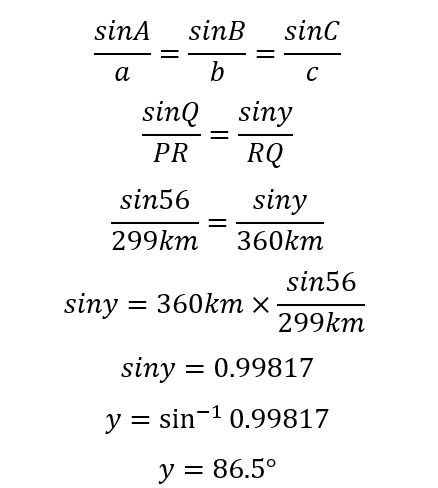cum
top of page
Search

# CSEC Mathematics: Bearings

A bearing is the angle (in degrees) measured from north in a clockwise direction. Bearings are always written using 3 digits, so, even when an acute angle is measured (clockwise from north, of course), such as 9°, the bearing would be written as 009°.

Questions regarding bearings always employ the use of another mathematical principle. For example: There are two points, A and B. If B is on a bearing of 62° from A, what is the bearing of A from B?

Diagrams are always useful in questions involving bearings- many questions in CSEC actually require that you draw a diagram to represent the information in the question.(Always remember to note the phrasing of the question, i.e. the bearing of A from B is the bearing you would measure from B in the direction of A)

Since we want to find the bearing of A from B, we need to calculate the value of the reflex angle (the outer angle) at B since bearings are always measured clockwise from north.

Looking at the diagram, you can probably tell that the two north lines (labelled N) are parallel cut by a transversal which connects points A and B. If you are unfamiliar with pairs of angles in parallel lines cut by transversals, please look at the following post for a quick walkthrough.

Anyway, we can find the smaller angle at B because it and 62° are co-interior angles. Subtracting 62° from 180° gives the value of the smaller angle at B, 118°. However, since we want to find the bearing (the angle on the outside), we subtract 118° from 360°, giving us 242°.This may seem a bit too convoluted, and that's because there is a far simpler way to do such questions.

If the bearing you know is less than 180° (so the point you want to find is on the right), you can simply add the bearing you know to 180°. Using the example above, this is because:

360°- (180°-62°)

360°-180°+62°

180°+62°

242°

If the bearing you know is more than 180° (so the point you want to find is on the left), you can simply subtract 180° from the bearing you know. Using the same example, if we were instead given the bearing of A from B (242°) and asked to find the bearing of B from A:

180°-(360°-242°)

180°-360°+242°

242°-180°

62°

This is because you would subtract 242° from 180° to find the angle co-interior to the angle you want to find. Subtracting that angle from 180° would give you your answer. However, subtracting 180° from the angle given is like a shortcut to the same answer.

Bearings questions rarely only involve two points, however. Most questions involving bearings will involve 3 points for which you are given certain bearings and distances. In the end, a triangle is formed between the three points, allowing you to use trigonometric ratios, cosine rule, sine rule and other rules relating to triangles to solve.

To show this, we will examine a question from CSEC Math Paper 2 2011 which tackles bearings:

The diagram below, not drawn to scale, shows the route of an aeroplane flying from Portcity (P) to Queenstown (Q) and then to Riversdale (R). The bearing of Q from P is 132° and the angle PQR is 56°.ii) The distance from Portcity (P) to Queenstown (Q) is 220 kilometres and the distance from Queenstown to Riversdale (R) is 360 kilometres. Calculate the distance RP.

Let's label the triangle with these distances to help us understand what's happening a bit better.Now we can see that we have the values of two sides and the angle between them. Do you remember which rule allows us to find the third side with this information? Cosine rule! (Read our post here if you are unfamiliar with cosine and sine rule)Using cosine rule, PR= 299.04 km.

iii) Determine the bearing of R from P.

If we observe the diagram, we will recognize that the bearing of Q from P (132°), when added to the apex angle of the triangle (P) will give us the bearing of R from P.Now that we know all three sides and the value of one angle is is possible for us to use either sine rule or cosine rule to calculate the value of y. To demonstrate both rules in this post, we will use sine rule.So, y=86.5°.

Bearing of R from P= 132°+86.5=218.5°

Bearing of R from P is 218.5°